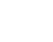# Reinforced Concrete Slab Design GuidelinesReading time: 1 minute

## Basics of Reinforced Concrete Slab Design

Slabs are generally designed on the assumption that they consists of a number of beams of breadth ‘one meter’.## 1. Effective Span of Slab

The effective span of a simply supported slab shall be taken as the lesser of the following:
1. Distance between the centers of bearings,
2. Clear span plus effective depth

## 2. Thickness of Slab

The following table gives the maximum values of the ratio of span to depth.
 Type of slab Ratio of span to depth Simply supported and spanning in one direction 30 Continuous and spanning in one direction 35 Simply supported and spanning in two directions 35 Continuous and spanning in two directions 40 Cantilever slabs 12

## 3. Reinforcement for Slab

Minimum reinforcement in either direction shall be 0.15 percent of total cross-sectional area. Main reinforcement which is based on the maximum bending moment shall not be less than 0.15 per cent of the gross sectional area. The pitch of the main bars shall not exceed the following:
1. Three times the effective depth of slab, and
2. 45 cm.
Distribution bars are running at right angles to the main reinforcement and the pitch shall not exceed
1. Five times the effective depth of slab, and
2. 45 cm.
The diameter of main bars may be from 8 mm to 14 mm. for distribution bars, steel 6 mm or 8 mm are generally used.

## 4. Reinforcement Cover

The minimum cover to outside of main bars shall not be less than the following:
1. 15 mm and
2. Diameter of the main bar.

## 5.  Concrete Slab Design Procedure

Steps to be followed in the design of slab
1. Assuming suitable bearings (not less than 10cm), find the span of the slab between the centers of bearings.
2. Assume the thickness of slab (take 4 cm per metre run of the span).
3. Find the effective span which is lesser of (i) distance between centres of bearings, and (ii) clear span and effective depth.
4. Find the dead load and the live load per square meter of the slab.
5. Determine the maximum bending moment for a one meter wide strip of the slab.
The maximum bending moment per meter width of slab,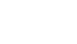Where, w = total load intensity per square meter of the slab.
1. Equate the balanced moment of resistance to the maximum bending moment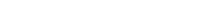Find the effective depth ‘d’ from the above equation.
1. Calculate the main reinforcement per metre width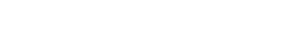For M15 concrete, lever arm = 0.87 d Spacing of bar =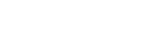## Design of Continuous Slab

Suppose a slab is supported at the ends and also at intermediate points on beams, the maximum sagging and hogging moments to which the slab is subjected to due to uniformly distributed load, can be computed as follows: Let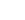= intensity of dead load per square metre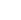= intensity of live load per square metre. Bending moment due to dead load and live load may be taken as follows (IS: 456 – 2000)
 Bending Moment At middle of end span Over support At middle of interior support Over interior support Bending Moment due to dead load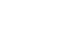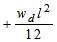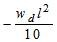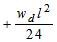Bending moment due to live load